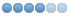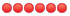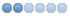# Debug help

• cunning
• Topic Author
• Offline
•2 months 1 week ago - 2 months 1 week ago #10260 by cunning
Debug help was created by cunning
I am having some issues with 2 main TBA functions ( i thnk this is just valgrind) but i figured i would ask. There is another that i may or may not get help with here.

valgrind --log-file="leak.txt" --leak-check=full --show-reachable=yes --track-origins=yes bin/circle_dev_w -q 6001
==848167== Memcheck, a memory error detector
==848167== Copyright (C) 2002-2017, and GNU GPL'd, by Julian Seward et al.
==848167== Using Valgrind-3.18.1 and LibVEX; rerun with -h for copyright info
==848167== Command: bin/circle_dev_w -q 6001
==848167== Parent PID: 840102
==848167==
==848167== Conditional jump or move depends on uninitialised value(s)
==848167==    by 0x1268B2: main (comm.c:285)
==848167==  Uninitialised value was created by a stack allocation

```char *fread_string(FILE *fl, const char *error)
{
char buf[MAX_STRING_LENGTH] = {'\0'}, tmp = {'\0'};
char *point = NULL;
int done = 0, length = 0, templength = 0;

*buf = '\0';
*tmp = '\0';

do
{
memset(tmp, '\0', 513);
if (!fgets(tmp, 512, fl))
{
log("SYSERR: fread_string: format error at or near %s", error);
exit(1);
}
/* If there is a '~', end the string; else put an "\r\n" over the '\n'. */
/* now only removes trailing ~'s -- Welcor */

point = strchr(tmp, '\0');

if (point == NULL)
{
log("String: %s", tmp);
exit(1);
}

[b] for (point--; (*point == '\r' || *point == '\n' || point == 0); point--)   ============> 3915[/b]
;

if (*point == '~')
{
*point = '\0';
done = 1;
}
else
{
*(++point) = '\r';
*(++point) = '\n';
*(++point) = '\0';
}```

==848167== Conditional jump or move depends on uninitialised value(s)
==848167==    by 0x1C6C71: boot_db (db.c:1122)
==848167==    by 0x126D48: init_game (comm.c:561)
==848167==    by 0x126D48: main (comm.c:395)
==848167==  Uninitialised value was created by a stack allocation

/* fread_clean_string is the same as fread_string, but skips preceding spaces */
```char *fread_clean_string(FILE *fl, const char *error)
{
char buf[MAX_STRING_LENGTH] = {'\0'}, tmp = {'\0'};
char *point = NULL, c = '\0';
int done = 0, length = 0, templength = 0;

*buf = '\0';
*tmp = '\0';

do
{
if (feof(fl))
{
return 0;
}
c = getc(fl);
} while (isspace(c));
ungetc(c, fl);

do
{
if (!fgets(tmp, 512, fl))
{
log("SYSERR: fread_clean_string: format error at or near %s", error);
exit(1);
}
/* If there is a '~', end the string; else put an "\r\n" over the '\n'. */
/* now only removes trailing ~'s -- Welcor */
point = strchr(tmp, '\0');
[b]  [/b] for (point--; (*point == '\r' || *point == '\n'); point--)  ==================>3982
;
if (*point == '~')
{
*point = '\0';
done = 1;
}
else
{
*(++point) = '\r';
*(++point) = '\n';
*(++point) = '\0';
}```

==848167== HEAP SUMMARY:
==848167==     in use at exit: 3,600,349 bytes in 2,677 blocks
==848167==   total heap usage: 2,109,239 allocs, 2,106,562 frees, 272,968,248 bytes allocated
==848167==
==848167== 5 bytes in 1 blocks are still reachable in loss record 1 of 369
==848167==    at 0x4848899: malloc (in /usr/libexec/valgrind/vgpreload_memcheck-amd64-linux.so)
==848167==    by 0x4BEC60E: strdup (strdup.c:42)
==848167==    by 0x4AE7B56: ??? (in /usr/lib/x86_64-linux-gnu/libmariadb.so.3)
==848167==    by 0x4AEC1DD: ??? (in /usr/lib/x86_64-linux-gnu/libmariadb.so.3)
==848167==    by 0x249B69: connect_to_mysql (mysql.c:62)
==848167==    by 0x1C68AB: boot_db (db.c:984)
==848167==    by 0x126D48: init_game (comm.c:561)
==848167==    by 0x126D48: main (comm.c:395)
```void connect_to_mysql()
{

if (mysql_library_init(0, NULL, NULL))        =================> 62
{
log("SYSERR: Unable to initialize MySQL library.");
exit(1);
}

if (!(conn = mysql_init(NULL)))
{
log("SYSERR: Unable to initialize MySQL connection.");
exit(1);
}

my_bool reconnect = 1;
mysql_options(conn, MYSQL_OPT_RECONNECT, &reconnect);

if (!mysql_real_connect(conn, MYSQL_SERVER, MYSQL_USER, MYSQL_PASSWD, MYSQL_DB, 0, NULL, 0))   ===========> 78 same as 62 debug
{
log("SYSERR: Unable to connect to MySQL: %s", mysql_error(conn));
exit(1);
}```
Last edit: 2 months 1 week ago by cunning.

• thomas
•• Offline
•2 months 1 week ago #10265 by thomas
Replied by thomas on topic Debug help
The two first ones are false positives. The variables in question are initialized in the same function, actually.

I must admit I know little about the C mysql-library, but this also looks like a false positive.

• cunning
• Topic Author
• Offline
•2 months 1 week ago - 2 months 1 week ago #10267 by cunning
Replied by cunning on topic Debug help
I thought so myself, but i definately have one that needs looked into.

==1124415== Invalid read of size 8
==1124415==    at 0x238C67: next_in_list (lists.c:548)
==1124415==    by 0x238C67: simple_list (lists.c:657)
==1124415==    by 0x238D97: free_list (lists.c:409)
==1124415==    by 0x126FB9: main (comm.c:419)
==1124415==  Address 0x5b78f48 is 8 bytes inside a block of size 24 free'd
==1124415==    at 0x484B27F: free (in /usr/libexec/valgrind/vgpreload_memcheck-amd64-linux.so)
==1124415==    by 0x238D8F: free_list (lists.c:410)
==1124415==    by 0x126FB9: main (comm.c:419)
==1124415==  Block was alloc'd at
==1124415==    at 0x484DA83: calloc (in /usr/libexec/valgrind/vgpreload_memcheck-amd64-linux.so)
==1124415==    by 0x2387BE: create_item (lists.c:370)
==1124415==    by 0x238863: create_list (lists.c:359)
==1124415==    by 0x1C6A8D: boot_db (db.c:1019)
==1124415==    by 0x126D68: init_game (comm.c:561)
==1124415==    by 0x126D68: main (comm.c:395)
==1124415==
==1124415==
==1124415== HEAP SUMMARY:
==1124415==     in use at exit: 329,281 bytes in 1,028 blocks
==1124415==   total heap usage: 3,009,434 allocs, 3,008,406 frees, 262,207,244 bytes allocated

```struct item_data *create_item(void)
{
struct item_data *pNewItem = NULL;

CREATE(pNewItem, struct item_data, 1);

pNewItem->pNextItem = NULL;
pNewItem->pPrevItem = NULL;
pNewItem->pContent = NULL;

return (pNewItem);
}```
```void free_list(struct list_data *pList)
{
void *pContent = NULL;

simple_list(NULL);

if (pList && pList->iSize)
while ((pContent = simple_list(pList)))   ========================> line 409
remove_from_list(pContent, pList);

/* Global List for debugging */
if (pList != global_lists)
remove_from_list(pList, global_lists);

free(pList);
}```
```void *next_in_list(struct iterator_data *pIterator)
{
void *pContent = NULL;
struct item_data *pTempItem = NULL;

if (pIterator->pList == NULL)
{
mudlog(NRM, ADMLVL_GOD, TRUE, "WARNING: Attempting to get content from iterator with NULL list.");
return NULL;
}

/* Cycle down the list */
pTempItem = pIterator->pItem->pNextItem;     ====================? line 548
pIterator->pItem = pTempItem;

/* Grab the content */
pContent = pIterator->pItem ? pIterator->pItem->pContent : NULL;

return (pContent);
}```
Last edit: 2 months 1 week ago by cunning.

• cunning
• Topic Author
• Offline
•2 months 1 week ago #10268 by cunning
Replied by cunning on topic Debug help
Just for the record, the mysql errors were me not closing the DB when we destroy_db() called. Leaving all those sql connections opened. It seriously reduced my error output by 700 lines.

• soth
• Offline
• Junior Member
•2 months 4 days ago #10276 by soth
Replied by soth on topic Debug help
Hi,
I do not use c with mysql, but I do with c# and one thing I learned back in 2005 was make sure you close and dispose of those connections when you are done with them :) Even though the garbage collector is supposed to clean up I usually use obj.Dispose() anyway.

I am just now starting to try and use a debugger for linux myself.

Cheers

• cunning
• Topic Author
• Offline
•